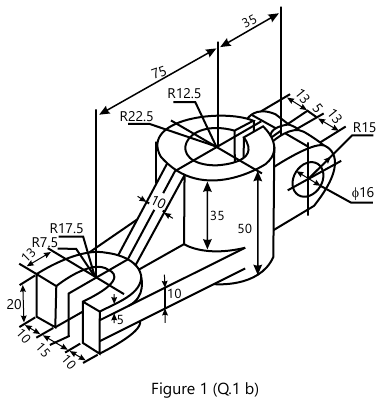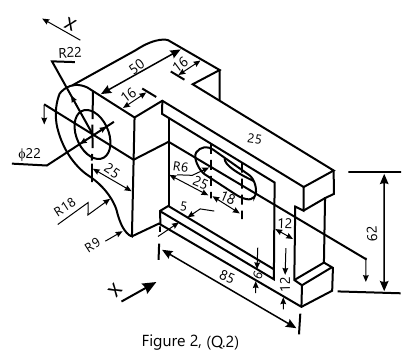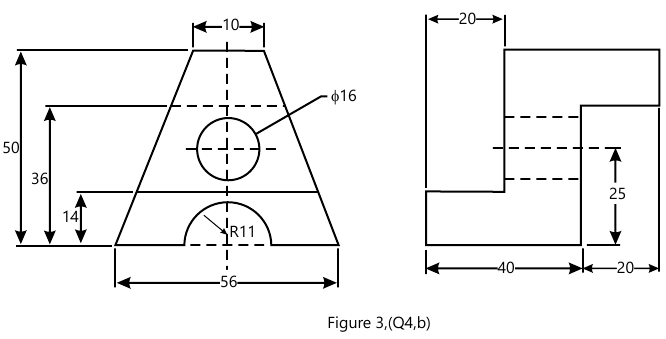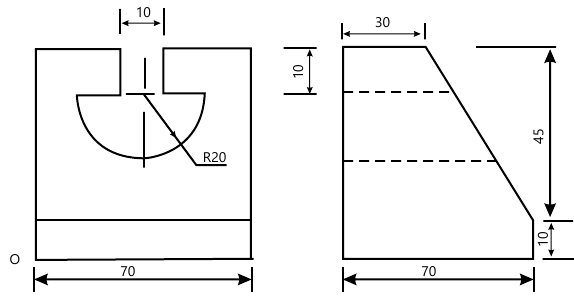MORE IN Engineering Drawing
MU First Year Engineering (Semester 2)
Engineering Drawing
May 2013
Total marks: --
Total time: --
INSTRUCTIONS
(1) Assume appropriate data and state your reasons
(2) Marks are given to the right of every question
(3) Draw neat diagrams wherever necessary

1 (a) A circular plate of diameter 60 mm rolls without slipping along a straight line inclined at 30° to horizontal. Draw locus of point of its contact with the line if it completes one rotation. Name the curve.
6 M
1 (b) For the given figure, draw
(i) FV.
(ii) TV.
Insert all dimensions.9 M

2 For the figure, draw
(i) FV.
(ii) Sectional TV.
(iii) RHS view.
Insert all dimensions.15 M

3 A pentagonal pyramid side of base 30 mm and axis 60 mm long stands on edge of base on HP. The edge makes an angle of 45° with the VP. Draw its projections if apex is 40 mm above HP and nearer to the observer.
15 M

4 (a) A cylindrical block of base diameter 80 mm and height 50 mm is resting on one of the base point on HP with axis inclined at 60° to HP. Draw its projections.
6 M
4 (b) For the given figure, draw isometric projection using natural scale.9 M

5 A right circular vertical cone, base diameter 50 mm and axis 60 mm long is cut by an AIP and bisecting the axis. Draw FV, Sectional TV and true shape of the section if true shape is an ellipse with major axis 40 mm. What is the inclination of the cutting plane with the HP? Also draw development of lateral surface of remaining portion of the solid.
15 M

6 (a) TV and FV of a line AB, 70 mm long measures 55 mm and 60 mm respectively. Draw its projections if end A is 15 mm above HP and 20 mm in front of VP. Determine its inclination with HP and VP.
9 M
6 (b) For the given figure, draw isometric projection using natural scale.6 M

More question papers from Engineering Drawing